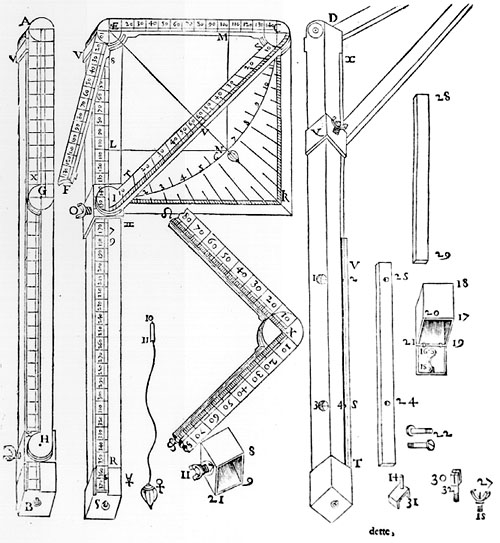Description AB: a board, about 72-cm-long (in box-wood or ebony, whose square section's side is 13 mm long. AG and GH are two metallic rules. Th AG e rule is hinged at A to a board and at G to a rule (GH), which is hinged to a square section's ring (H). At the centre of the page the instrument is shown: ES is the board, FIC is the Balestrino, forming a right angle IEC; the ring IO is blocked in its place by means of a screw (O), EF is the other leg. Both the square's parts CE and EF and the leg ES are divided into tens each divided again into ten degrees. The tens' length is as long as the length of the radius of the circle where the rules' heads are embedded together, as is shown in X. The diagonal CI is used as a quadrant. In order to show the degrees on it, a quarter of the circle whose radius is the same as EC must be drawn on a sheet of paper, and it must be divided into 120 degrees, 60 per each part. By leaning the instrument on the drawing, divisions are drawn on the diagonal: in this way, the sixth degree from the two sides starting from the centre N is obtained. This is used by bombers to focus the artillery at the elevation required to hit the target; the elevation on the diagonal CI can be read with the aid of a plumb-line, blocked into E. Onto the back part of the two rules (ECI) two lines are drawn with the balls' diameters and respective weights. Another line is drawn along a rule and its distance from the first line will be useful to know the wind required per each ball. The diameter of a one-pound ball is drawn on it as well. By measuring this distance with a compass, the square ring is moved along the ES leg until the oblique distance between the two points shown on the two lines is found, then it is blocked at the right point by the screw O. After that, the instrument will show all the balls' weights and winds.